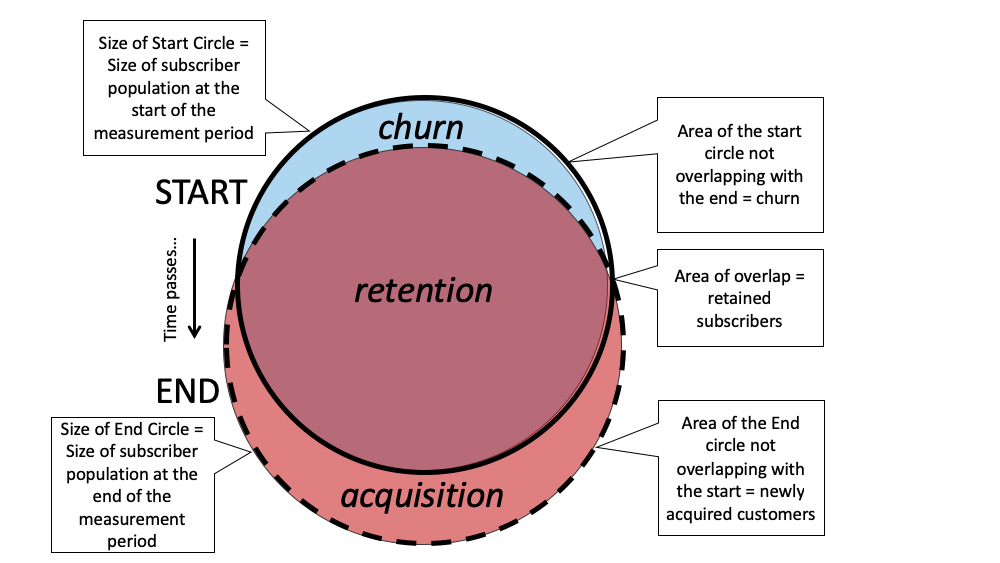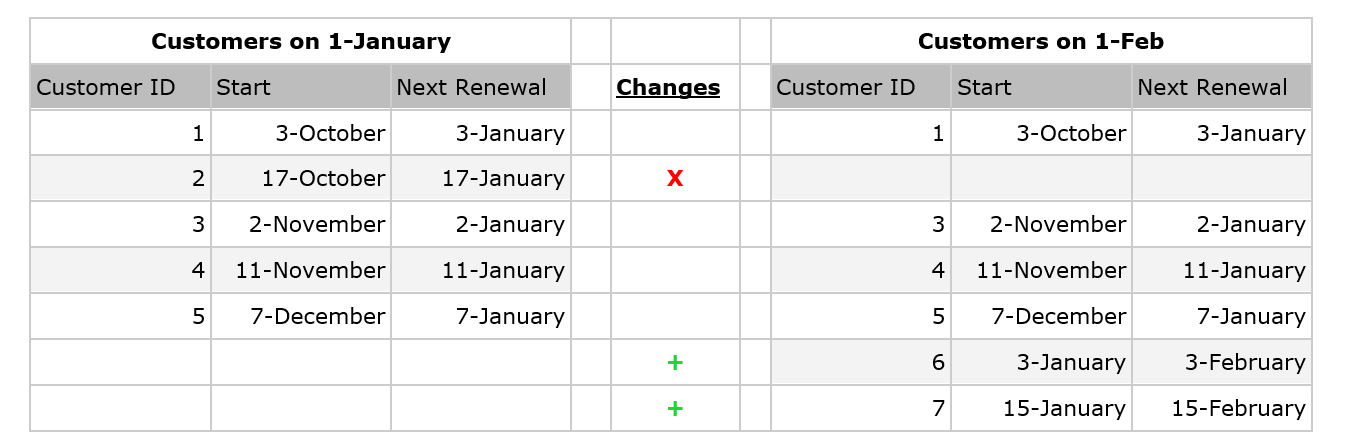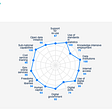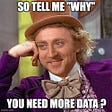# The Meaning of the Churn Rate

From Fighting Churn with Data by Carl Gold

__________________________________________________________________

Take 37% off Fighting Churn with Data by entering fccgold into the discount code box at checkout at manning.com.
__________________________________________________________________

## The Meaning of the Churn Rate

Let’s start out with a visualization. Figure 1 demonstrates the idea of churn in a diagram. Each of the two circles represents the subscriber pool at different points in time. The area of the circle can represent either the number of subscribers or the total amount that they pay, the latter being used whenever subscribers pay different amounts. Whether churn is based on the number of subscribers or the amount of revenue, the concept’s the same. The churn is the downward facing crescent at the top, the part of the start circle which isn’t overlapping the bottom (end) circle — which represents those subscribers who are no longer with the service. To complete the picture: the overlap between the two circles are the retained subscribers, and the upward crescent at the bottom of the end circle which doesn’t overlap the top circle are the newly acquired subscribers. Note that in general the size of the two circles won’t be exactly the same, and Figure 1 shows a growing subscription service where acquisition is larger than churn.Figure 1. Churn Illustration: Two overlapping circles illustrate the concept of churn. Each circle represents the size of the customer base at a different point in time. In the example, the circle for the end (lower circle) is larger, indicating a growing service. The overlap between the two circles are retained customers, the downward crescent which is left out at the end is churn. The upward crescent that wasn’t part of the start represents newly acquired customers.

The churn rate is defined as the proportion of the start subscribers have left by the end time. In an equation this is:

Where “Start” in Equation 1 means the area of the start circle, and “Churn” means the area of the “Churn” crescent. Figure A shows a simple example of this, assuming the product has only five customers on January 1st. All customers have a monthly renewal, on the same day of the month as they started. During the month of January, one customer doesn’t renew, and two new customers sign up such that by February 1st there are six customers. The churn rate measured over the month of January is:Figure 2. A Churn Calculation Example: Simplified example of a churn rate calculation. The customers on January 1st are shown on the left and customers on February 1st are shown on the right. Every customer renews every month, on the same date as they signed up. Over the month of January, one customer doesn’t renew (a churn, shown by the “X”) and there are two new customers who sign up (shown by the “+”). The churn rate is the number who churned (1) divided by the number who were on the service at the start (5), or 1/5=80%. Note that the two new signups don’t influence the churn calculation.

Note that the churn rate doesn’t use either the total subscribers at the end, or the subscribers acquired. Look at the diagram to understand why: the churn is the portion of the start circle that isn’t covered by the end circle, but the size of the end circle depends on both retention and the amount of new subscriber acquisition. The acquisition of new subscribers is an incredibly important subject but it’s a separate matter from the churns because it results from a different set of processes (and it’s normally the job of different departments to manage). Consequently, the churn rate is based only on churn in relation to the subscribers at the start; if the churn were instead divided by the area of the End subscriber pool it’d be incoherent because it’d be mixing the parts of the two pools and confusing the contributions of acquisition and retention to the size of the subscriber pool.

Similarly, the retention rate is defined in Equation 2 as:

Considering the example in Figure A the retention rate’s:

Finally, considering the parts of the Start circle it’s clear that

If you divide both sides of Equation 3 by the Start subscribers you get:

By substituting the definitions from Equation 1 and Equation 2 produces the relationship:

or,

Equation 4 is easy to understand considering Figure 1: Churn and Retention together make up the start circle, and the sum of the two proportions must add up to the whole circle, or 100%.

TAKEAWAY: The retention rate can easily be calculated from the churn rate, and visa-versa. Which measurement you look at is kind of a matter of preference.

Most organizations use the churn rate for internal discussions around reducing churn; the retention rate is usually used to report to outsiders (e.g. investors) when the emphasis is meant to be positive (“the glass is half full”).

## The Standard (Account) Churn Rate

This section describes the standard churn rate, which is the churn rate based on subscriber counts.

DEFINITION: Churn measurement based on subscriber count’s referred to as the “standard” churn or “the churn”.

This is the simplest churn rate in its meaning because it’s unaffected by upsells, downsells and expiration of discounts. It always refers to the proportion of subscribers that completely cancel off of the service. The standard churn rate is often called the “account churn rate” because it refers to the complete churn of account holders, who may hold multiple subscriptions. For the standard churn rate, an account holder who cancels one subscription but still keeps another subscription isn’t considered a churn (this is considered a downsell). In the B2B space, the standard churn rate is also referred to as “Logo Churn” because each account holder is a company, or a “logo”. I’ll demonstrate how to calculate the churn rate directly, rather than calculating it from retention; direct calculation requires the SQL feature known as Outer Joins. It’s true that the churn rate could be calculated from account retention using an inner join like the one used for Net Retention, but being able to identify churned accounts with an outer join’s a skill you’ll need later. Because outer joins aren’t a basic SQL feature I’ll review outer joins as I explain the query.

## Standard Churn Rate Definition

Before considering the outer join, let’s first review the steps to take in order to calculate the standard churn rate, in relation to Figure 1 and Equation 1.

1. Set the start and end times for the measurement
2. Identify the subscribers at the start and count the total number of them; this is the top circle in Figure 1
3. Identify the subscribers at the end; this is the bottom circle in Figure 1
4. Identify the churned subscribers and count how many there are: this is the upper (downward facing) crescent in Figure 1
5. Divide the number of churns by the number of accounts at the start. This is Equation 1

## Outer Joins for Churn Calculation

The churned accounts are selected from the start and end accounts using an SQL feature called an outer join. I’ll review outer joins briefly for readers who aren’t already familiar. An inner join is the more common type of join, like the join on account_id to create the retained_accounts CTE in Figure 2: It returns all matching rows (according to the join field) and returns the desired fields from both tables for the matches. An outer join is different because instead of only returning the matches it returns all the rows from one table. The join returns matching rows from another table as usual, but it fills fields from the second table with NULLS for non-matching rows (i.e. account_Id’s which are in the start_accounts but not the end_accounts get NULL for the end account_id). This is known as a “left outer join” because all the rows from the first table are selected, which is on the left hand side of the join statement. A “right outer join” behaves in the opposite way as far as which table keeps all its rows, and a “full outer join” returns all rows from both tables. Understanding left outer joins suffices for churn.

An outer join is used to find the churned accounts because the point is to find the accounts present at the start which aren’t present at the end, and if you do an inner join those are precisely the ones to remove. A left outer join returns all the accounts present at the start and not only the ones who churned and this is why the retained accounts CTE also includes a where clause: It selects only those accounts from the join where the account_id on the end is NULL, meaning it chooses only those rows from the join which were in the start_accounts CTE for which the matching account_id isn’t in the end_account CTE.

TAKEAWAY: Outer joins can be used to find rows which don’t match a join criteria, making it useful for identifying churns.

Look back at the churn diagram in Figure 1 because the figure also provides an illustration of the logic of inner and outer joins. The retained accounts are the intersection of the start and end accounts, which are selected by an inner join. The churned accounts, the left outer join, are found from the starting accounts by removing all those which have a match at the end with the where clause selecting for NULL end account_id. It follows that the acquired accounts would be the right outer join with a where clause selecting for NULL start account_id, this is left as an exercise for the reader.

## Standard Churn Calculation SQL

The SQL for the program is shown in Listing 1. It’s identical to the net retention query from Listing 1 for the first three CTEs, in which it creates temporary tables containing the accounts for the start and end of the measurement. But after finding the accounts it creates a table of churned accounts, rather than a table of retained accounts.

Listing 1 Standard (Account) Churn SQL Program

`with date_range as ( #A       select  '2017-01-01'::date as start_date, '2018-01-01'::date as end_date  ), start_accounts as #B (       select distinct account_id    #C          from subscription s inner join date_range d on             s.start_date <= d.start_date   #D             and (s.end_date > d.start_date or s.end_date is null) ), end_accounts as #E (       select distinct account_id  #P       from subscription s inner join date_range d on             s.start_date <= d.end_date   #D             and (s.end_date > d.end_date or s.end_date is null) ), churned_accounts as #G (       Select s.account_id       from start_accounts s       left outer join end_accounts e on #H             s.account_id=e.account_id  #I       where e.account_id is null    #J ), start_count as (  #K       select    count(start_accounts.*) as n_start from start_accounts ), churn_count as ( #L       select    count(churned_accounts.*) as n_churn from churned_accounts ) select       n_churn::float/n_start::float as churn_rate, #M       1.0-n_churn::float/n_start::float as retention_rate, #N       n_start, #O       N_churn #O from start_count, churn_count`

#A This sets the time period for which the SQL calculates churn.

#B This CTE contains all of the accounts ids active at the start.

#C Use a distinct query because you can’t assume that every account has only one subscription.

#D The criteria for being active on a given date: The start date is on or before the date, and the end date’s after the date or null.

#E This CTE contains all of the account ids active at the end.

#G This CTE contains all of the account ids for accounts that churned: those which were active at the start but inactive at the end.

#H The outer join includes all the records at the start, because it’s a left outer join.

#I The join looks for records that match by account id, and fills with Nulls for the end accounts where there’s no match.

#J Removes records where there was no churn because they have a non-null e.account_id. Only those accounts which were in the start_accounts CTE but not in the end_accounts CTE remain.

#K Count the number which were active at the start.

#L Count the number of churned accounts.

#M The churn rate formula: Number churned / Number at start

#N The retention rate formula : 1-churn rate

#O Print these to show how the churn rate was produced.

The following describes each CTE in the program and the final select statement, and what role it plays in the calculation:

1. date_range : A one row table holding the start and end date for the calculation. This is step 1.
2. start_accounts : A table with one row per account active at the start. This table is created by selecting from the subscription table based on the condition that the account’s active at the start of the churn measurement. This is step 2.
3. end_accounts : A table with one row per account active at the end of the churn measurement. The condition for being “active’ is the same as for the start accounts, using the churn measurement end date for the criteria. This is step 3.
4. churned_accounts : A table with one row per account which is active at the start but inactive at the end. This is created by the outer join on account id between the start_accounts table and the end_accounts table, and the where clause which removes accounts if the end account_id isn’t NULL. This is step 4.
5. start_count : A one row table that sums the total number of accounts at the start of the churn measurement, for clarity
6. churn_count : A one row table that sums the total number of accounts that churned during the measurement period, for clarity
7. The final select statemen : it takes the results from the one row result tables start_count and churn_count and calculates the final results by plugging the values into Equations 1 and Equations 2. This is step 5, the final step in the program.

This shows that in a series of five steps the Churn rate and Retention rate described by equations 1 and 2 can be calculated from a typical subscription database in SQL. This SQL program was tested on a sample data set loaded into a Postgres 8 database and produced the result show in Figure 4.

## When to use the Standard Churn Rate Calculation

The standard churn rate is used as the main operational metric whenever all subscribers pay similar amounts or the subscription’s free. If all subscriber pay exactly the same (meaning no discounts or any variation, or the product]s free) standard churn can be calculated with either a net retention query or the standard churn rate query. If there]s some amount of pricing variation, or if there are temporary discounts, then you should use the standard churn calculation method in this section. The standard churn rate has a special role in churn analysis, because churn analysis uses a model designed to predict customer (subscription holder) churn, a correctly calibrated predictive churn model should reproduce the standard account churn rate. For multi-price subscriptions or those with extensive use of discounts the standard churn rate isn’t the best churn rate as an operational metric — for those scenarios you should use MRR Churn.

TAKEAWAY: If there’s a moderate amount of pricing variation, including discounts, then you should use the standard churn calculation method in this section. Moderate pricing variation is when the most valuable customers pays as much as around twice the least valuable customers.

--

--

--

## More from Manning Publications

Love podcasts or audiobooks? Learn on the go with our new app.

## Who Drives the total Experience in a Modern Company?## 2019 Market report: SaaS consultant’s guide to implementation focus## Wisdom from Above the Clouds## We are not American entrepreneurs 🇺🇸## Charities are Facing an Existential Threat: Themselves## 5 things to keep in mind when starting a Craft Brewery in 2020## Less Is More## Steve’s ITK: You’re in good company## The Global Data Barometer first edition Released — Part 1## Stacked Generalisation theory and implementation.## 3-Step Data-lite Machine Learning